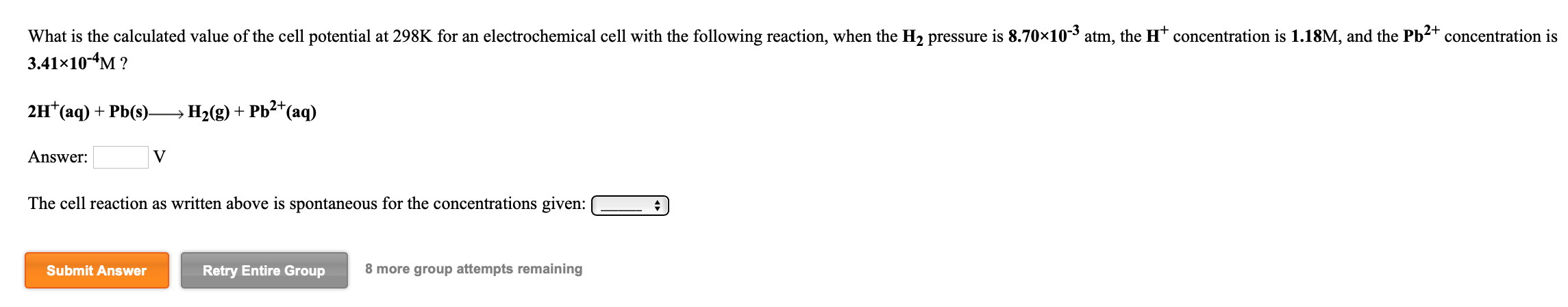# What is the calculated value of the cell potential at 298K for an electrochemical cell with the following reaction, when the H2 pressure is 8.70×10-3 atm, the H+ concentration is 1.18M, and the Pb2+ concentration is 3.41×10-4M ? 2H+(aq) + Pb(s) H2(g) + Pb2+(aq) Answer: ____V The cell reaction as written above is spontaneous for the concentrations given: true/false